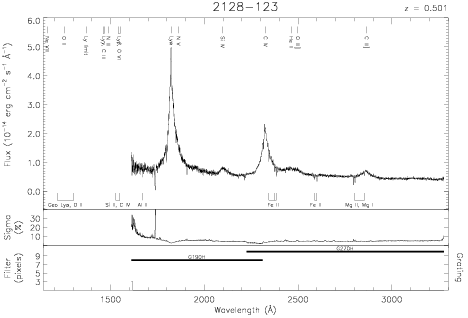Download dataset
TELESCOP= 'HST     '
INSTRUME= 'FOS     '
RADECSYS= 'FK5     '
EQUINOX =               2000.0
DATE-OBS= '1993-11-02T05:39:18'
RA_PNT  =       322.8975824814
DEC_PNT =       -12.1181948417
PA_PNT  =       170.1710968018
OBJECT  = '2128-123'
ROOTNM01= 'Y1HE0F03T'
GRNDMD01= 'RAPID-READOUT'
DETECT01= 'AMBER   '
APERID01= 'C-2     '
FGWAID01= 'H19     '
ROOTNM02= 'Y1HE0F04T'
GRNDMD02= 'RAPID-READOUT'
DETECT02= 'AMBER   '
APERID02= 'C-2     '
FGWAID02= 'H19     '
ROOTNM03= 'Y1HE0F05T'
GRNDMD03= 'RAPID-READOUT'
DETECT03= 'AMBER   '
APERID03= 'C-2     '
FGWAID03= 'H19     '
ROOTNM04= 'Y1HE0F06T'
GRNDMD04= 'RAPID-READOUT'
DETECT04= 'AMBER   '
APERID04= 'C-2     '
FGWAID04= 'H27     '
ROOTNM05= 'Y1HE0F07T'
GRNDMD05= 'RAPID-READOUT'
DETECT05= 'AMBER   '
APERID05= 'C-2     '
FGWAID05= 'H27     '